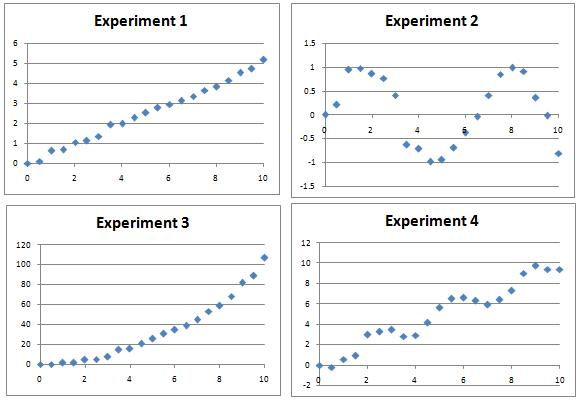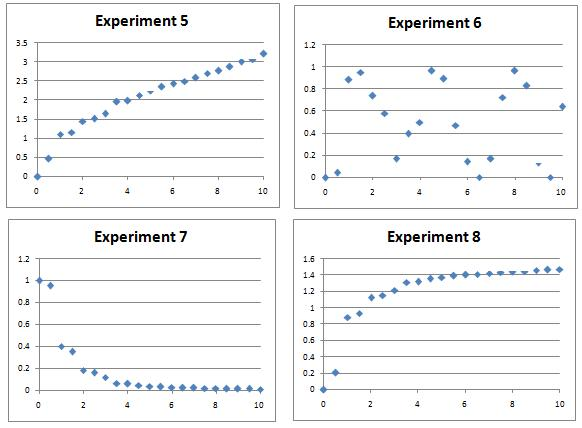### Submit a Resource

NRICH: Back Fitter

10 graphs of experimental data are given. Can you use a spreadsheet to find algebraic graphs which match them closely, and thus discover the formulae most likely to govern the underlying processes?

Several experiments were performed and data measured over a period of 10 hours.

The resulting charts are shown below.

Can you find algebraic equations which closely match the curves, which could be used to predict values of the variables at other times? There might be many possible curves of the right sort of shape by eye, so a numerical plot will be needed to discover the most likely candidates.

The following Office 2003 spreadsheet will allow you easily to compare plots of the likely curves against the actual data – the intention is that you will tackle this problem numerically.

Note: The different sets of experimental data are distinct, so try as many or as few as you like. Fitting all of the sets will present quite a challenge!Extension: Whilst there are ‘obvious’ candidates for each data set, can you find multiple functions which give rise to apparently good matches to some of the data sets? How might you numerically determine which fits are best?

Age 14 to 16

Math Topics
Algebra & Pre-Algebra, Functions & Graphs
High School, Educator

## Organization

NRICH (University of Cambridge)

Challenge